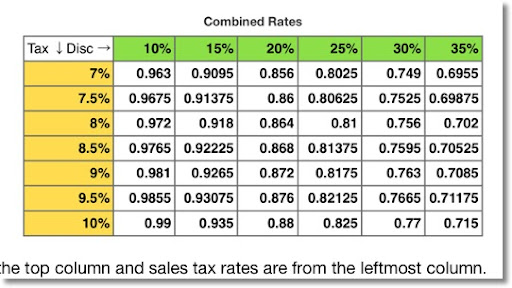## Sunday, July 28, 2013

### Discounts and Sales Tax: What is the Final Price?

SALE! GET THIS WONDERFUL ITEM FOR A SUBSTANTIAL DISCOUNT! GOOD FOR THIS WEEKEND ONLY!

Chances are that we have all seen this advertisement at least one time in our lives. Chances are that if you are shopping with someone, that person asks what the price is after the discount. And in a lot of instances, depending on where you live, you have to take sales tax into consideration. Today's blog is about how to find the final price after the discount and sales tax.

Note: All the examples presented here are using prices stated in dollars (\$). Rest assured the concepts hold for all other units of currency. Also, all dollar amounts are rounded to two decimal places.

The Math Behind it All

Discounts

Let D be the discount. Since the discount is customarily stated as a percentage, such as "take 20% off of everything on this rack", we'll express the discount as its decimal equivalent: 0.01*D. Discounts are subtracted from the price.

For example, a item normally sold for \$59.99 which has a 20% discount has a discounted price of \$47.99.

\$59.99 - 20%
\$59.99 - \$59.99 * 0.01 * 20
\$59.99 * (1 - 0.20)
\$59.99 * 0.80
\$47.99

A general formula for subtracting D% from X is:

X - D%
X - X * 0.01 * D
X * (1 - 0.01 * D)

Sales Tax

Let S be the sales tax. Sales tax works like discounts, except that sales tax is added to the price. We'll express the sales tax as its decimal equivalent: 0.01*S.

Where I live, which is located in the County of Los Angeles in California, the sales tax is 9%. For example, if I purchase a taxable item for \$59.99, taking sales tax into account, the total cost to me is \$65.39.

\$59.99 + 9%
\$59.99 + \$59.99 * 0.01 * 9
\$59.99 + \$5.40
\$65.39

Likewise, we can use a general formula for adding S% to X is:

X + S%
X + 0.01 * S * X
X * (1 + 0.01 * S)

The Final Price of a Discounted Item After Sales Tax

Let's come up with a general formula where we determine the final price of an item which normally sells for \$P (or whatever currency you work with), sold at a discount D%, which the item subject to sales tax of S%.

Customarily, the discount gets applied before the sales tax. Then the final price works out to be:

\$P - D% + S%

(\$P - D%) + S%
(\$P - \$P * 0.01 * D) + S%
(\$P * (1 - 0.01 * D) + S%

(\$P * (1 - 0.01 * D) + (\$P * (1 - 0.01 * D)) * S * 0.01
(\$P * (1 - 0.01*D))(1 + 0.01*S)

So the final cost is:
\$P * (1 - 0.01*D) * (1 + 0.01*S)

Taking from our example before, let's say that the item originally priced at \$59.99 is discounted at 20%. What is the final cost after 9% sales tax is applied?

P = \$59.99
D = 20
S = 9
And the final cost is:

\$59.99 * (1 - 0.01*20) * (1 + 0.01*9) = \$52.31

In the next section, we will determine a "combined rate".

Simplified Rate Considering Both Discount and Sales Tax

Here we are going to find a general formula for a "combined rate", or a rate that takes both the discount and sales tax rate into account.

Basically, the "combined rate" is what the final price would be if the original price was \$1.00. (\$P = \$1.00)

The combined rate is (1 - 0.01D)*(1+0.01S)

In our example the combined rate for an item discounted 20%, subject to sales tax of 9% is 0.872. (87.2%)

(1 - 0.01*20)(1 + 0.01*9)
0.872

Under these conditions, you should expect to pay 87.2% of the original price when it is all said and done. For an item with an original price of \$59.99, the final cost is:

Price * Combined Rate
\$59.99 * 0.872
\$52.31

Another example: item has an original price of \$18.99. The discount is 10% while the sales tax rate is 9%

Combined rate:
(1 - 0.10 * 10) * (1 + 0.01 * 9)
0.981

Final cost:
\$18.99 * 0.981
\$18.63

As a check, let's find the final cost if we applied the discount then sales tax step by step.
(\$18.99 - 10%) + 9%
(\$18.99 - \$18.99 * 0.01 * 10) + 9%
\$17.09 + 9%
\$17.09 + \$17.09 * 0.01 * 9
\$18.63

It checks out.

This is a quick table of combined rates using various sales tax and discount rates. The iOS App Numbers is used to generate this table.Example: What is the final cost for at item originally priced \$69.95 that is discounted 35%. The sales tax rate is 10%.

From the table above, the combined rate is 0.715. The final cost is \$69.95 * 0.715 = \$50.01.

I want to do another mathematical series. I plan to do a short series of ordinary differential equations within the next couple of months, but if there is anything you suggest, subjects, calculators, calculator programming languages, please do. Many thanks as always.

My next blog entry will be within a couple of weeks. I am taking care of family and also going on a short vacation next weekend - hopefully the weather at Landers, CA will be nice as I go on my first astronomical star party.

Eddie

This blog is property of Edward Shore. 2013

### Casio fx-CG50: Sparse Matrix Builder

Casio fx-CG50: Sparse Matrix Builder Introduction The programs can create a sparse matrix, a matrix where most of the entries have zero valu...